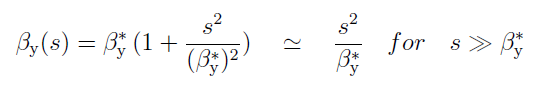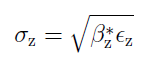# What does the hourglass factor H measure?

Around an IP the transverse beam size grows as the distance to the IP (focus) increases. The betatron function evolves in the drift to the first quadrupole as (where s is the distance from the IP) - here example for the vertical plane (for hor. plane replace y by x):Since the beam size is given by (z=x,y, epsilon is the emittance):The beam size grows roughly like s between IP and first quadrupole.

When the betatron function b* at the IP (beta measures the beam envelope and is defined by the optics) is large compared to the bunch length, to a very good approximation the beam size is constant along the length of the colliding bunch (case of LEP beta* 5 cm for bunch length of 1 cm, and current LHC beta* of 60 cm for bunch length of 9 cm). For FCC-ee bunch length (2 mm) and beta* (1 mm) become comparable, as a consequence the beam size varies along the colliding bunch. Since head and tail have larger sizes, the luminosity is reduced as compared to the case of constant beam size. The H factor measures this loss of luminosity.

Category: